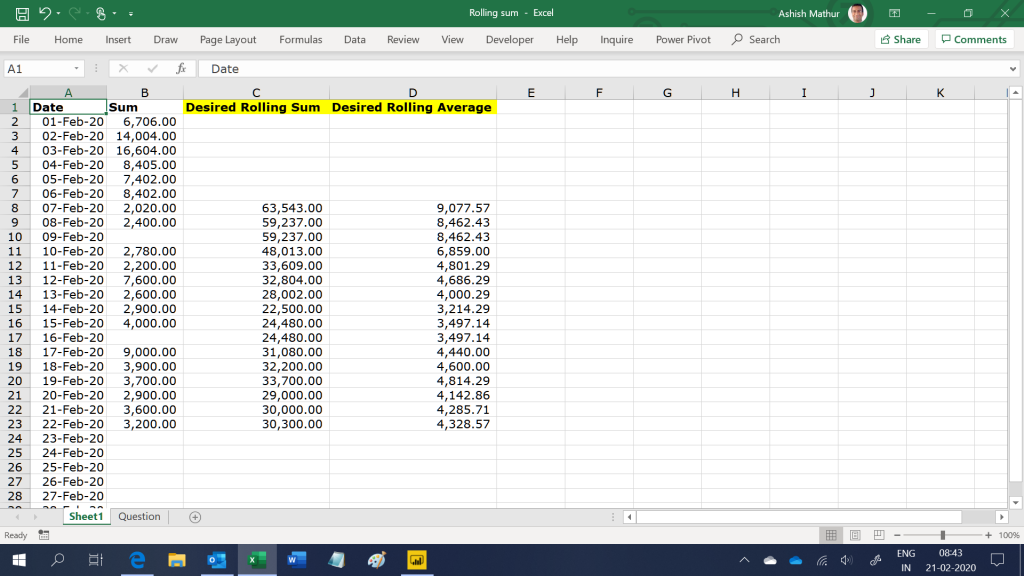Assume a simple dataset as shown in the image below (the input data is in columns A and B only.  The desired outcome is in columns C and D).The objective is to calculate the 7 days rolling sum and average (as shown in columns C and D) ignoring blank cells.  So in cell C8, the rolling sum is the summation of values from range B2:B8.  In cell C9, it is from B3:B9.  However, in cell C10, it will be from range B3:B9 (not from range B4:B10).  Likewise, in cell C11, the rolling sum will be from range B4:B11.  So the range to be considered for calculating the rolling sum has to roll back automatically until it picks up 7 numeric cells – the blanks have to be ignored.  The rolling average is a simple division – Rolling sum/7.

I have solved this question with Excel formulas here.  This time however, I am sharing a solution by using the DAX formula language available in the PowerPivot and PowerBI Desktop.  You may download my PowerBI Desktop file from here.  The same solution can also be obtained in MS Excel using the PowerPivot as well.